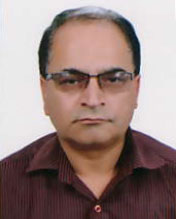Message from HODMathematics is one of the oldest and well developed disciplines of basic sciences. It plays an important role in physical, biological, engineering management and social sciences as well. We claim that Mathematics is Everywhere. Due to emerging technologies and advanced sciences, growing research int...
Master's Level

Course Structures

The department conducts only masters’ level in Kirtipur campus. Here, the students come from the different affiliated colleges with the Tribhuvan University. The department unashamedly pursues a curriculum in pure and basic mathematics, and it deals with its subject matter with considerable rigor. Depth rather than breadth has been the hallmark of the education here; and all this with the belief that anyone sufficiently versed in the rigorous techniques of mathematics can successfully move on to more applied and even to neighboring fields. This, we believe, is a more sensible way to make use of the very limited amount of high level human power of the country.

The last fifty years have witnessed a dramatic upgrading of the level of education throughout the country, and mathematics education is no exception, Materials taught not long ago at the master and bachelors level have permeated down to the certificate level, and some even to the 9th and 10th grades in school. This, and also the introduction of the three-year bachelor program prompted a full-scale revision of the curriculum at the master level, which the department has carried out with various measures of success.

Now the department is conducting four semesters program for the master level students, following the four years program of the bachelor (undergraduate) level program. The present courses for the two-year/ four semester master's program are:

Math 551 Topology I

Math 552 Topology II

Math 553 Measure Theory and Integration I

Math 554 Functional Analysis I

Math 555 Complex Analysis and Applications I

Math 556 Differential Equations

Math 557 Theory of Functions I

Math 558 Theory of Functions II

Math 559 Differential Geometry

Math 560 Combinatorial Optimization

Math 561 Mathematical Programming

Math 562 Mathematical Analysis I

Math 563 Functions of Several Variables

Math 564 Elementary Harmonic Analysis

Math 565 Algebra I

Math 566 Algebra II

Math 567 Linear Algebra

Math 568 Linear Algebra and Its Applications

Math 569 Introduction to the Theory of Sets

Math 570 Theory of Numbers I

Math 571 Mechanics

Math 572 Functional Analysis II

Math 573 Harmonic Analysis

Math 574 Special Functions I

Math 575 Special Functions II

Math 576 Partial Differential Equations I

Math 577 Integral Transforms I

Math 578 Measure Theory and Integration II

Math 579 Scheduling Theory

Math 580 Integer Programming

Math 581 Network Optimization

Math 582 Graph Theory

Math 583 Numerical Analysis

Math 584 Numerical Optimization

Math 585 Dynamical Systems

Math 586 Dynamics of Viscous Fluids

Math 587 Partial Differential Equations II

Math 588 Introduction to C Programming

Math 589 Seminar

Math 590 Thesis

Math 591 Integral Transforms II

Math 592 Complex Analysis and Applications II

Math 593 Theory of Numbers II

Math 595 Analysis of finite difference schemes